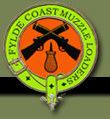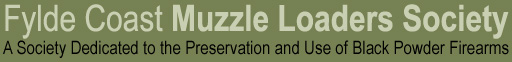Let's Do the Twist! A Guide to Calculating the Ideal Bullet Length [A handy formula discovered on the Internet by Keith Dale] Here is a handy formula for calculating ideal bullet length: L = 150 x CAL x CAL / T ..where L is length of bullet in inches, CAL is calibre in decimals of an inch and T is rate of twist of the rifling in inches. * Note that this formula becomes less accurate at the larger calibres used in black powder firearms